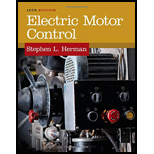# The rotating magnetic field of a two-pole motor travels 360 electrical degrees. How many mechanical degrees does the rotor travel?### Electric Motor Control

10th Edition
Herman
Publisher: CENGAGE L
ISBN: 9781133702818

#### Solutions

Chapter
Section### Electric Motor Control

10th Edition
Herman
Publisher: CENGAGE L
ISBN: 9781133702818
Chapter 32, Problem 1SQ
Textbook Problem
117 views

## The rotating magnetic field of a two-pole motor travels 360 electrical degrees. How many mechanical degrees does the rotor travel?

To determine

Mention the mechanical degrees at which the two-pole motor travels for 360 electrical degree.

The motor travels at 360 mechanical degrees.

### Explanation of Solution

Given data:

The electrical degree is θe=360°.

The number of poles in the system P is 2.

Formula used:

Write the general relationship between mechanical and electrical degree.

θm=θe(2P) (1)

Here,

P is the number of poles,

θm is the mechanical degree, and

θe is the electrical degree.

Substitute 2 for P and 360° for θe in Equation (1) to find the mechanical degree θm.

θm=360°(22)θm=360°

Conclusion:

Thus, the motor travels at 360 mechanical degrees.

#### The Solution to Your Study Problems

Bartleby provides explanations to thousands of textbook problems written by our experts, many with advanced degrees!

Get Started

Find more solutions based on key concepts
For Problem 11.17, determine the thermal conductivity of the unknown sample for the set of data given in accomp...

Engineering Fundamentals: An Introduction to Engineering (MindTap Course List)

How is an incident response plan different from a disaster recovery plan?

Principles of Information Security (MindTap Course List)

Calculate the magnitudes of the pin reactions at A and C. Neglect the weights of the members.

International Edition---engineering Mechanics: Statics, 4th Edition

What is the smallest graduation on a metric micrometer with a vernier scale?

Precision Machining Technology (MindTap Course List)

How does using a proxy server help protect your online identity? (66)

Enhanced Discovering Computers 2017 (Shelly Cashman Series) (MindTap Course List)

Why is it important to not weld when everything is wet?

Welding: Principles and Applications (MindTap Course List)

If your motherboard supports ECC DDR3 memory, can you substitute non-ECC DDR3 memory?

A+ Guide to Hardware (Standalone Book) (MindTap Course List)